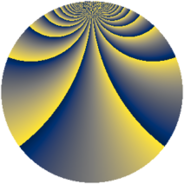# Properties

 Label 1840.2.crLevel $1840$ Weight $2$ Character orbit 1840.cr Rep. character $\chi_{1840}(127,\cdot)$ Character field $\Q(\zeta_{44})$ Dimension $1440$ Sturm bound $576$

# Related objects

## Defining parameters

 Level: $$N$$ $$=$$ $$1840 = 2^{4} \cdot 5 \cdot 23$$ Weight: $$k$$ $$=$$ $$2$$ Character orbit: $$[\chi]$$ $$=$$ 1840.cr (of order $$44$$ and degree $$20$$) Character conductor: $$\operatorname{cond}(\chi)$$ $$=$$ $$460$$ Character field: $$\Q(\zeta_{44})$$ Sturm bound: $$576$$

## Dimensions

The following table gives the dimensions of various subspaces of $$M_{2}(1840, [\chi])$$.

Total New Old
Modular forms 6000 1440 4560
Cusp forms 5520 1440 4080
Eisenstein series 480 0 480

## Trace form

 $$1440q + O(q^{10})$$ $$1440q + 48q^{21} - 48q^{41} + 48q^{53} + 96q^{57} + 96q^{65} - 48q^{77} + 96q^{81} + 216q^{85} + 48q^{93} + O(q^{100})$$

## Decomposition of $$S_{2}^{\mathrm{new}}(1840, [\chi])$$ into newform subspaces

The newforms in this space have not yet been added to the LMFDB.

## Decomposition of $$S_{2}^{\mathrm{old}}(1840, [\chi])$$ into lower level spaces

$$S_{2}^{\mathrm{old}}(1840, [\chi]) \cong$$ $$S_{2}^{\mathrm{new}}(460, [\chi])$$$$^{\oplus 3}$$